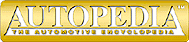The Physics of Racing
Part 8: Simulating Car Dynamics with a Computer Program

These articles were written by Brian Beckman (brianbec@microsoft.com) physicist, and member of No Bucks Racing Club. ©Copyright 1991, Brian Beckman

This month, we begin writing a computer program to simulate the physics of racing. Such a program is quite an ambitious one. A simple racing video game, such as ``Pole Position,'' probably took an expert programmer several months to write. A big, realistic game like ``Hard Drivin''' probably took three to five people more than a year to create. The point is that the topic of writing a racing simulation is one that we will have to revisit many times in these articles, assuming your patience holds out. There are many `just physics' topics still to cover too, such as springs and dampers, transients, and thermodynamics. Your author hopes you will find the computer programming topic an enjoyable sideline and is interested, as always, in your feedback.

We will use a computer programming language called Scheme. You have probably encountered BASIC, a language that is very common on personal computers. Scheme is like BASIC in that it is interactive. An interactive computer language is the right kind to use when inventing a program as you go along. Scheme is better than BASIC, however, because it is a good deal simpler and also more powerful and modern. Scheme is available for most PCs at very modest cost (MIT Press has published a book and diskette with Scheme for IBM compatibles for about \$40; I have a free version for Macintoshes). I will explain everything we need to know about Scheme as we go along. Although I assume little or no knowledge about computer programming on your part, we will ultimately learn some very advanced things.

The first thing we need to do is create a data structure that contains the mathematical state of the car at any time. This data structure is a block of computer memory. As simulated time progresses, mathematical operations performed on the data structure simulate the physics. We create a new instance of this data structure by typing the following on the computer keyboard at the Scheme prompt:

```
(new-race-car)```
This is an example of an expression. The expression includes the parentheses. When it is typed in, it is evaluated immediately. When we say that Scheme is an interactive programming language, we mean that it evaluates expressions immediately. Later on, I show how we define this expression. It is by defining such expressions that we write our simulation program.

Everything in Scheme is an expression (that's why Scheme is simple). Every expression has a value. The value of the expression above is the new data structure itself. We need to give the new data structure a name so we can refer to it in later expressions:

```
(define car-161 (new-race-car))```
This expression illustrates two Scheme features. The first is that expressions can contain sub-expressions inside them. The inside expressions are called nested. Scheme figures out which expressions are nested by counting parentheses. It is partly by nesting expressions that we build up the complexity needed to simulate racing. The second feature is the use of the special Scheme word define. This causes the immediately following word to become a stand-in synonym for the value just after. The technical name for such a stand-in synonym is variable. Thus, the expression car-161, wherever it appears after the define expression, is a synonym for the data structure created by the nested expression (new-race-car).

We will have another data structure (with the same format) for car-240, another for car-70, and so on. We get to choose these names to be almost anything we like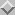. So, we would create all the data structures for the cars in our simulation with expressions like the following:

```
(define car-161 (new-race-car))
(define car-240 (new-race-car))
(define car-70  (new-race-car))```

The state of a race car consists of several numbers describing the physics of the car. First, there is the car's position. Imagine a map of the course. Every position on the map is denoted by a pair of coordinates,and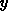. For elevation changes, we add a height coordinate,. The position of the center of gravity of a car at any time is denoted with expressions such as the following:

```
(race-car-x car-161)
(race-car-y car-161)
(race-car-z car-161)```

Each of these expressions performs data retrieval on the data structure car-161. The value of the first expression is thecoordinate of the car, etc. Normally, when running the Scheme interpreter, typing an expression simply causes its value to be printed, so we would see the car position coordinates printed out as we typed. We could also store these positions in another block of computer memory for further manipulations, or we could specify various mathematical operations to be performed on them.

The next pieces of state information are the three components of the car's velocity. When the car is going in any direction on the course, we can ask ``how fast is it going in thedirection, ignoring its motion in theanddirections?'' Similarly, we want to know how fast it is going in thedirection, ignoring theanddirections, and so on. Decomposing an object's velocity into separate components along the principal coordinate directions is necessary for computation. The technique was originated by the French mathematician Descartes, and Newton found that the motion in each direction can be analyzed independently of the motions in the other directions at right angles to the first direction.

The velocity of our race car is retrieved via the following expressions:

```
(race-car-vx car-161)
(race-car-vy car-161)
(race-car-vz car-161)```

To end this month's article, we show how velocity is computed. Suppose we retrieve the position of the car at simulated time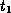and save it in some variables, as follows:

```
(define x1 (race-car-x car-161))
(define y1 (race-car-y car-161))
(define z1 (race-car-z car-161))```
and again, at a slightly later instant of simulated time,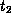:
```
(define x2 (race-car-x car-161))
(define y2 (race-car-y car-161))
(define z2 (race-car-z car-161))```
We have used define to create some new variables that now have the values of the car's positions at two times. To calculate the average velocity of the car between the two times and store it in some more variables, we evaluate the following expressions:
```
(define vx (/ (- x2 x1) (- t2 t1)))
(define vy (/ (- y2 y1) (- t2 t1)))
(define vz (/ (- z2 z1) (- t2 t1)))```
The nesting of expressions is one level deeper than we have seen heretofore, but these expressions can be easily analyzed. Since they all have the same form, it suffices to explain just one of them. First of all, the define operation works as before, just creating the variable vx and assigning it the value of the following expression. This expression is
```
(/ (- x2 x1) (- t2 t1))```
In normal mathematical notation, this expression would read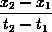and in most computer languages, it would look like this:
```
(x2 - x1) / (t2 - t1)```
We can immediately see this is the velocity in thedirection: a change in position divided by the corresponding change in time. The Scheme version of this expression looks a little strange, but there is a good reason for it: consistency. Scheme requires that all operations, including everyday mathematical ones, appear in the first position in a parenthesized expression, immediately after the left parenthesis. Although consistency makes mathematical expressions look strange, the payback is simplicity: all expressions have the same form. If Scheme had one notation for mathematical expressions and another notation for non-mathematical expressions, like most computer languages, it would be more complicated. Incidentally, Scheme's notation is called Polish notation. Perhaps you have been exposed to Hewlett-Packard calculators, which use reverse Polish, in in which the operator always appears in the last position. Same idea, and advantages, as Scheme, only reversed.

So, to analyze the expression completely, it is a division expression

```
(/ ...)```
whose two arguments are nested subtraction expressions
```
(- ...) (- ...)```
The whole expression has the form
```
(/ (- ...) (- ...))```
which, when the variables are filled in, is
```
(/ (- x2 x1) (- t2 t1))```
After a little practice, Scheme's style for mathematics becomes second nature and the advantages of consistent notation pay off in the long run.

Finally, we should like to store the velocity values in our data structure. We do so as follows:

```
(set-race-car-vx! car-161 vx)
(set-race-car-vy! car-161 vy)
(set-race-car-vz! car-161 vz)```
The set operations change the values in the data structure named car-161. The exclamation point at the end of the names of these operations doesn't do anything special. It's just a Scheme idiom for operations that change data structures.